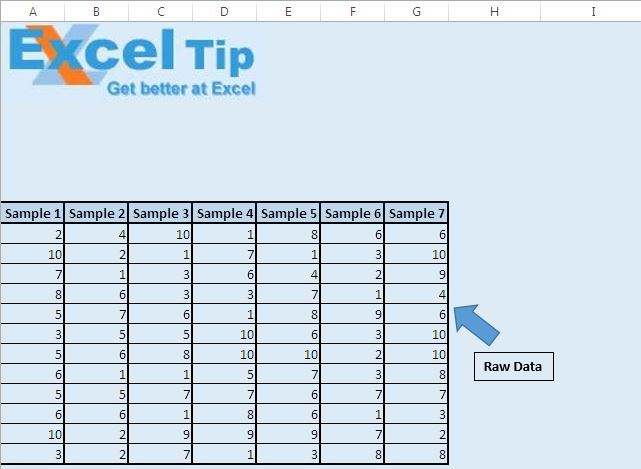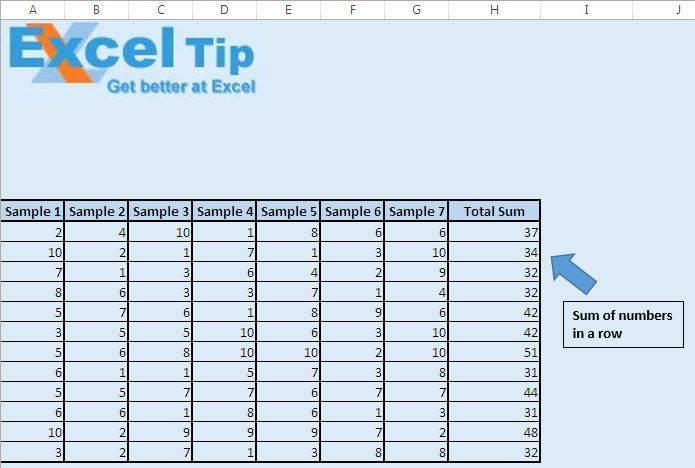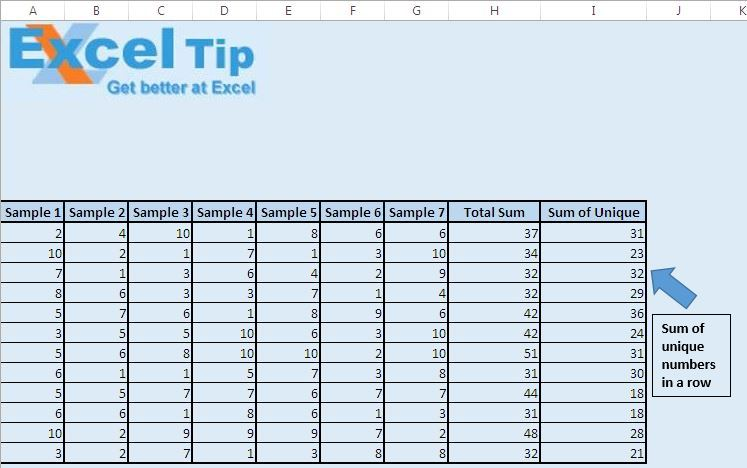# Add unique values using VBA in Microsoft Excel

In this article, we will create a User Defined Function (UDF) or custom function to add unique values in a defined range.

Raw data for this article consists of random numbers in seven different samples.Add another column to find the sum of values in each row.“Total Sum” column displays the sum of numbers in each row.

Now, add another column to find the sum of only unique numbers in each row. We have created a User Defined Function “AddDistinctValues” to find the sum of unique numbers. Add formula =AddDistinctValues(A11:G11) in Cell I11 and drag the formula to other rows.

UDF “AddDistinctValues” will give the sum of unique numbers in the defined range. In the image below, one can easily see the difference between the total sum and the sum of unique numbers.Logic explanation

UDF “AddDistinctValues” takes range object as input. It adds up unique numbers in the input range.

To find unique numbers in the input range, we have used collection object. As we know collection cannot store duplicate values, when we insert numbers in the input range in the collection, duplicate numbers won't be re-inserted in the collection, instead an error will be generated.

For handling the error, we have used “On Error Resume Next” statement. This statement won’t halt the function on encountering the error, instead Loop will move to the next statement.

Once we have the unique numbers in the collection, we can add them in array.

```
Function AddDistinctValues(InputRange As Range) As Integer

'Declaring variables
Dim Rng As Range
Dim UniqueValues As New Collection
Dim UniqueValue As Variant

'Recalculates the function whenever calculation occur in any other cell
Application.Volatile

On Error Resume Next

'Adding unique values to collection from input range
For Each Rng In InputRange
Next

On Error GoTo 0

For Each UniqueValue In UniqueValues
Next

End Function

```